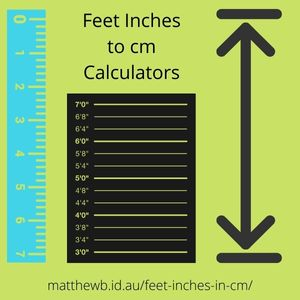## 5 feet 0 inches in cm Calculator

Enter feet in the top textbox. Enter inches in the 2nd textbox. Click Get Results button. Result appear below in cm.

Inputs
feet
inches
cm

FeetInchescm
3091
3193
3296
3399
34101
35104
36106
37109
38111
39114
310116
311119
40121
41124
42127
43129
44132
45134
46137
47139
48142
49144
410147
411149
50152
51154
52157
53160
54162
55165
56167
57170
58172
59175
510177
511180
60182
61185
62187
63190
64193
65195
66198
67200
68203
69205
610208
611210

## 5 feet 0 inches in cm FAQ

### How many centimeters are there in one inch?

There are 2.54 centimeters in one inch.

### How do you convert 5 feet and 0 inches into centimeters?

Use the feet inches in cm above or use the feet inches in cm table above.
5 feet and 0 inches = 152 cm.

### How many centimeters in 5'0"?

5'0" = 152 cm. Use the feet inches in cm calculator above.

### How many centimeters is 5 foot 0 inches?

There are 152 centimeters in 5 foot 0 inches. Use the feet inches in cm converter above.

### What is 5 feet 0 inches in cm?

5 feet 0 inches equals 152 cm. Use the feet inches in cm conversion calculator above.